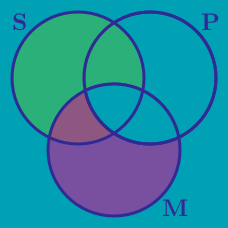Logic

# Basic Syllogism Logic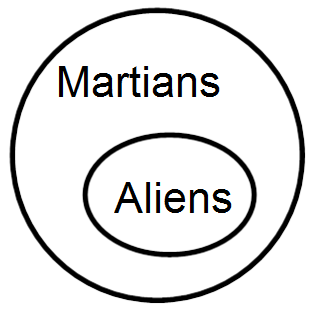Based on the diagram above, which of these statements is true?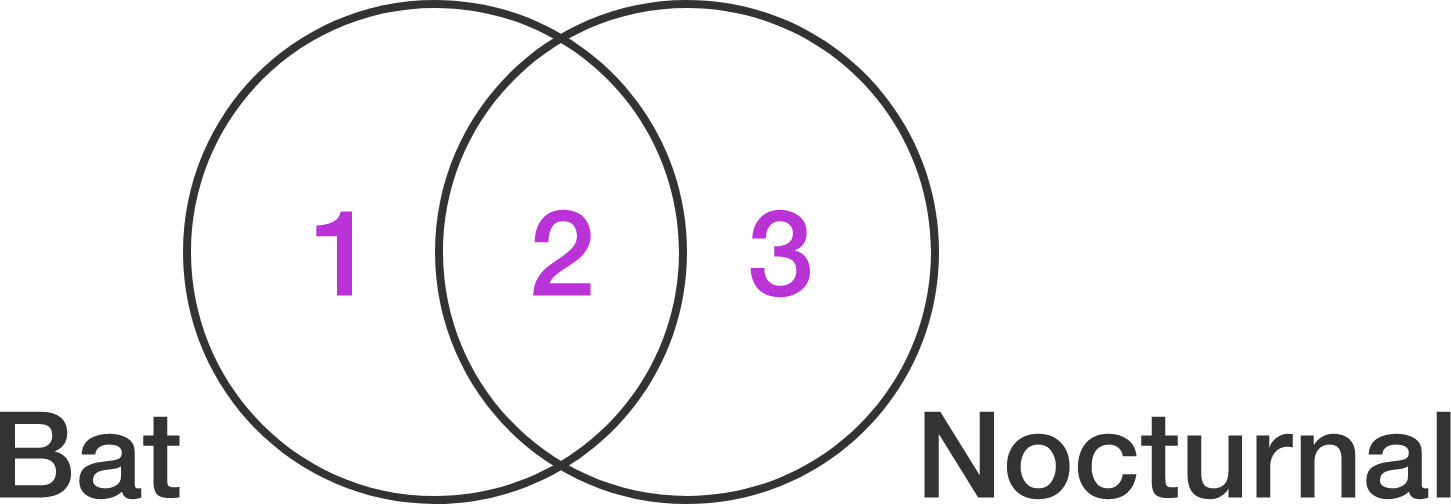If the statement "all bats are nocturnal" is represented on the diagram above, which region of the diagram will be empty? (Conventional definitions for the words used in these problems do not apply).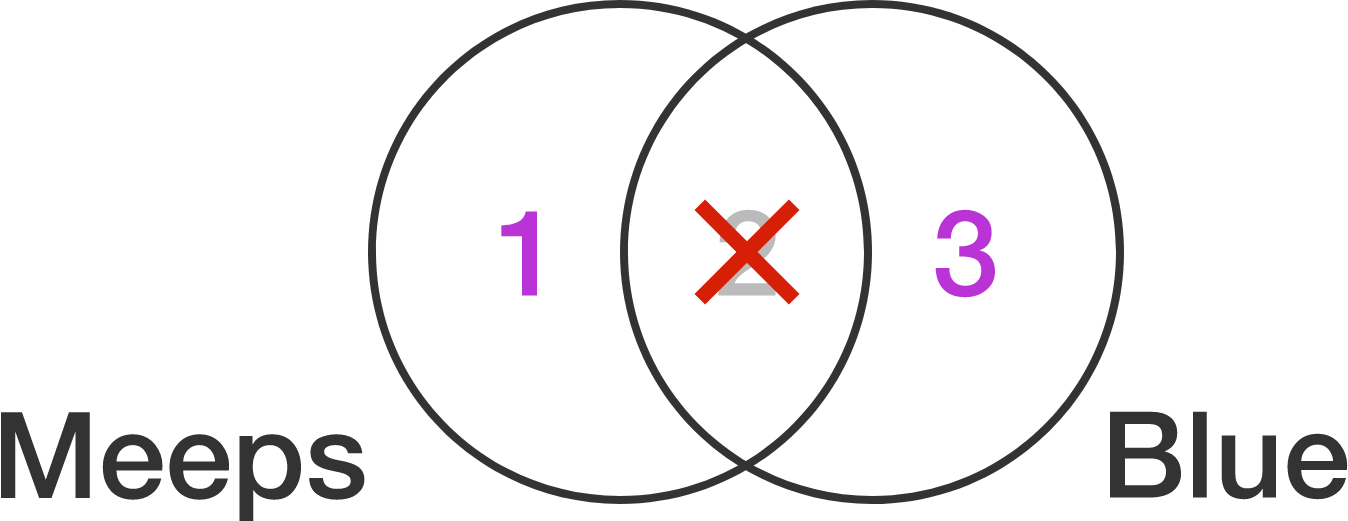The intersection between Meeps and Blue is crossed out; nothing is in that section. This means $\text{\_\_\_\_\_\_\_\_\_\_}.$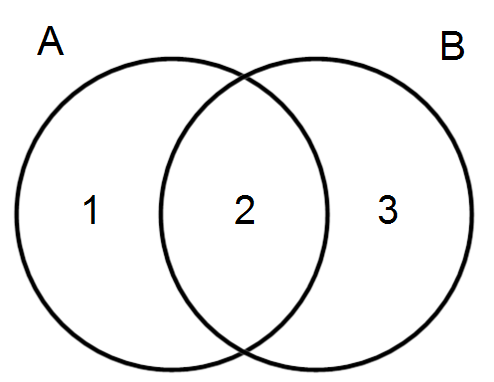The statement "some A are B" means which section of the diagram above can be crossed out because it will empty?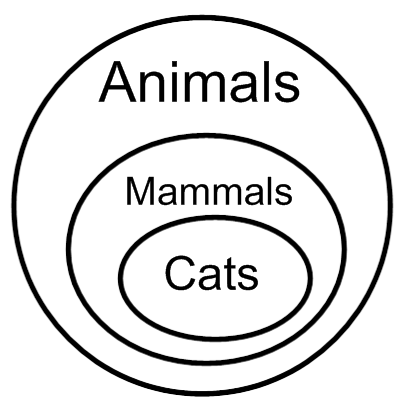Based on the diagram, which statement is true?

×